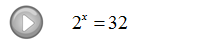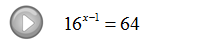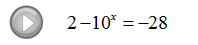## Algebra

Showing posts with label logarithm. Show all posts
Showing posts with label logarithm. Show all posts

### Solving Logarithmic Equations

Use the one-to-one property for logarithms to solve logarithmic equations.

If we are given an equation with a logarithm of the same base on both sides we may simply equate the arguments.

Step 1: Use the rules of exponents to isolate a logarithmic expression (with the same base) on both sides of the equation.
Step 2: Set the arguments equal to each other.
Step 3: Solve the resulting equation.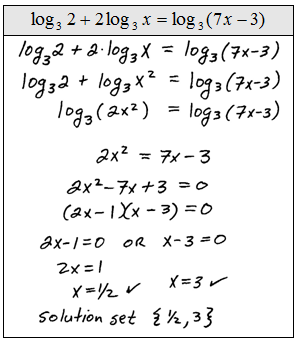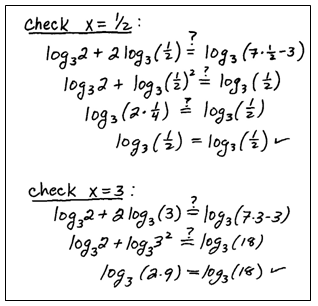Be sure to check to see if the solutions that you obtain solve the original logarithmic equation. In this study guide we will put a check mark next to the solution after we determine that it really does solve the equation. This process sometimes results in extraneous solutions so we must check our answers.
Solve.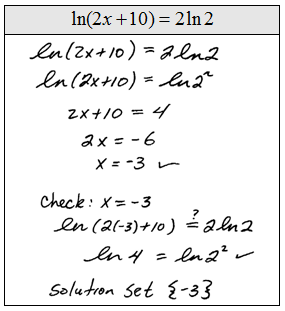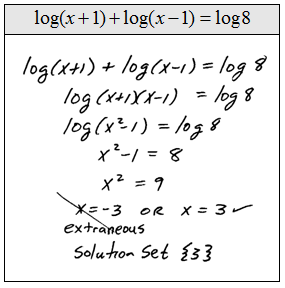Of course, equations like these are very special.  Most of the problems that we will encounter will not have a logarithm on both sides. The steps for solving them follow.

Step 1: Use the properties of the logarithm to isolate the log on one side.
Step 2: Apply the definition of the logarithm and rewrite it as an exponential equation.
Step 3: Solve the resulting equation.If the answer to the logarithmic equation makes the argument negative then it is extraneous.  This does not preclude negative answers. We must be sure to check all of our solutions.

Instructional Video: Solving Logarithmic Equations

Solve.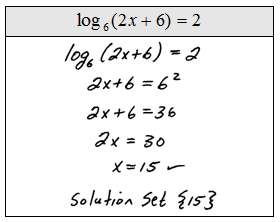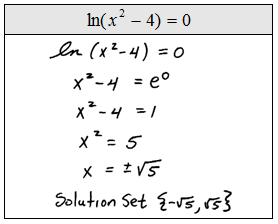Tip:  Not all negative solutions are extraneous!  Look at the previous set of problems and see that some have negative answers. The check mark indicates that we actually plugged the answers in to see that they do indeed solve the original.  Please do not skip this step, extraneous solutions occur often.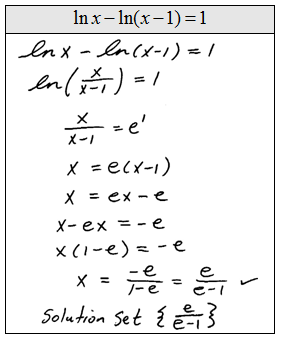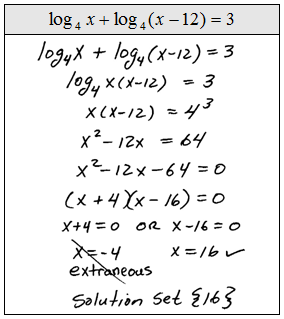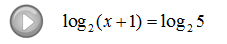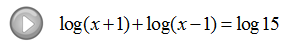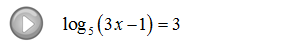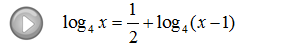### Solving Exponential Equations

In this section, we will make use of what we have learned about exponential functions to solve equations.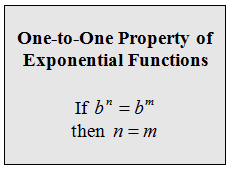Make use of the above property if you are able to express both sides of the equation in terms of the same base.

Step 1: Express both sides in terms of the same base.
Step 2: Equate the exponents.
Step 3: Solve the resulting equation.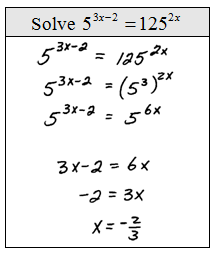Solve.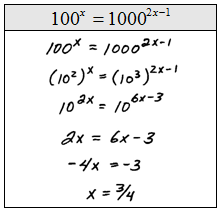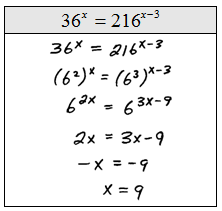It is not always the case that we will be able to express both sides of an equation in terms of the same base.  For this reason we will make use of the following property.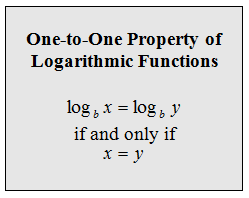Make use of the above property if you are unable to express both sides of the equation in terms of the same base.

Step 1: Isolate the exponential and then apply the logarithm to both sides.
Step 2: Apply the power rule for logarithms and write the exponent as a factor of the base.
Step 3: Solve the resulting equation.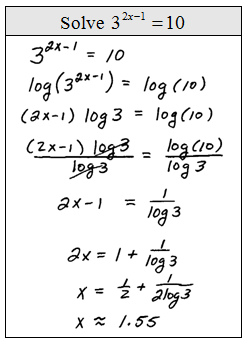Solve.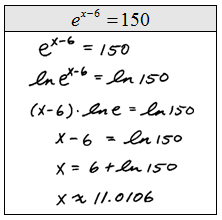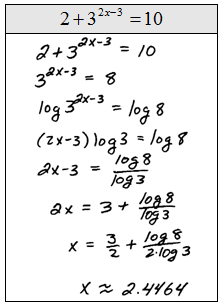When solving exponential equations and using the above process, the rule of thumb is to choose the common logarithm unless the equation involves the natural base e.   We choose these because there is a button for them on the calculator. However, we could certainly choose any base that we wish; this is the basis for the derivation of the change of base formula.